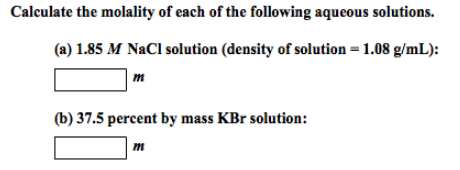# Problem: Calculate the molality of each of the following aqueous solutions. (a) 1.85 M NaCl solution (density of solution = 1.08 g/mL): (b) 37.5 percent by mass KBr solution:

###### FREE Expert Solution
93% (485 ratings)###### Problem Details

Calculate the molality of each of the following aqueous solutions.

(a) 1.85 M NaCl solution (density of solution = 1.08 g/mL):

(b) 37.5 percent by mass KBr solution: